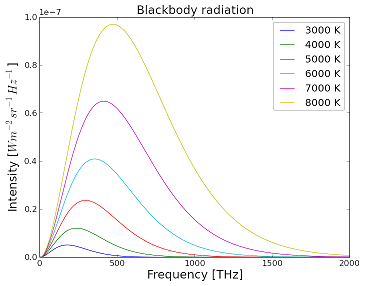Figure 1. Blackbody radiation curves for six different temperatures.

Blackbody radiation, sometimes called cavity radiation, refers to the behavior of a system that absorbs all radiation that is incident upon it and then re-radiates energy. This re-radiated energy is characteristic of the system and doesn't depend on the energy that is hitting it. The radiated energy depends strongly on the temperature of the object instead. The object on which the radiation is incident is a special type of object known as a blackbody, a theoretically ideal radiator and absorber, which absorbs all radiation that is incident upon it.

While there's no such thing as a perfect blackbody, most solid objects are sufficiently close to being a blackbody that they can be treated as being 'close enough'. The sun giving off sunlight, for example, can be treated as a perfect blackbody. On the other hand, the Earth needs to have a correction factor. This correction is taken to be a percentage, 70% of a perfect blackbody. This means that the radiation emitted still looks like figure 1, but it is 30% less energy, more or less uniformly across all of the wavelengths. The Earth's atmosphere cannot be treated as a blackbody. Please see solar energy to the Earth for a more detailed discussion of this.

It is difficult to imagine an object that absorbs and emits all wavelengths with equal probability but not equal magnitude. In other words the object would be equally capable of giving off any wavelength of light, it just wouldn't tend to because different wavelengths have different energies. The way physicists often imagine this object is as a hollow metal box with a very small hole in it. This box is in thermal equilibrium and is filled with radiation that bounces around inside the box, being emitted and reflected by the metallic walls. The tiny hole in one wall of the box allows some of the radiation from in the box to escape. Radiation from outside the box hits the hole and is trapped inside the box and is not likely to be re-emitted from the tiny hole, thus no reflections occur from the blackbody. The only radiation that emerges from the hole is a sample of the radiation bouncing around inside the box.

## The Ultraviolet Catastrophe

In classical physics, the predictions were that an ideal blackbody at thermal equilibrium would emit radiation with infinite power. This came from another law, Rayleigh-Jeans Law, that expresses energy as being proportional to . This law predicted that the sum of all the energies at all wavelengths resulted in an infinite amount of energy in the system. The term "ultraviolet catastrophe" was used to refer to this paradox.

Physicist Max Planck resolved this paradox by predicting that energy came in discrete amounts, known as quanta. This is a main principle in quantum mechanics. The equation he derived was not proportional to any longer, but rather took the form:

where is the frequency of the energy, is Planck's constant, is the temperature in Kelvin, and is Boltzmann's constant. For this equation, when the frequency is low the average energy follows the classical predictions, but at higher frequencies the average energy tends to zero.

These blackbodies closely approximate real world objects that are in thermodynamic equilibrium, meaning that they have no net energy flow since the flow in and out has the same rate. As the temperature of the blackbody changes, the curve adjusts and shifts so that the emitted curve balances the absorbed energy. From these curves, you can tell what color or wavelength of light is being emitted by the blackbody. This is done by looking for the peak wavelength using Wien's displacement law:

From this and Figure 1 it can be seen that hotter blackbodies emit their peak energies at shorter wavelengths. For blackbodies, both the wavelength at which the body radiates most strongly at - the peak wavelength, found using Wien's Law - and the total amount of radiation of the blackbody, depends on its temperature. The total energy radiated by a blackbody is determined from the area of the curve, and the actual value can be determined from the Stefan-Boltzmann law. As the temperature increases, the total radiation given off by the blackbody increases while the wavelength at which this radiation peaks at becomes smaller (more white or blue light is given off). This dependence on temperature is why stove burners glow red and the Sun glows orange while different stars at a different temperature may have a different colour.